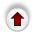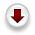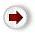# 4. Recursion As A Programming Technique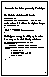Let us now turn to the final way in which you might use or encounter recursion in computer science. Almost all programming languages allow recursive functions calls. That is they allow a function to call itself. And some languages allow recursive definitions of data structures. This means we can directly implement the recursive definitions and recursive algorithms that we have just been discussing.

For example, the definition of the factorial function

```        factorial(0) = 1
factorial(n) = n * factorial(n-1)   [ for n > 0 ].```

can be written in C without the slightest change:

```int factorial(int n)
{
if (n == 0) return 1 ;
else        return n * factorial(n-1) ;
}```

Similarly, our strategy for adding up the values in array can be implemented directly in C. Here is the strategy:

• if FIRST=LAST, the answer is X[FIRST].
• if FIRST+1=LAST, the answer is X[FIRST] + X[LAST]
• Otherwise, choose any M between FIRST and LAST, and:
• add up the numbers in X between positions FIRST and M; the answer is S1 ;
• add up the numbers in X between positions M+1 and LAST; the answer is S2
• the final answer is S1 + S2.

Here is the C code:

```int addup(int first, int last, int x[])
{
int s1, s2, m ;
if (first   == last) return x[first];
else if (first+1 == last) return x[first] + x[last];
else {
m  = first + 1 ;
s1 = addup( first,    m, x);
s2 = addup(   m+1, last, x);
return s1 + s2;
}
}```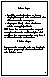The main benefits of using recursion as a programming technique are these:

• invariably recursive functions are clearer, simpler, shorter, and easier to understand than their non-recursive counterparts.

• the program directly reflects the abstract solution strategy (algorithm).
From a practical software engineering point of view these are important benefits, greatly enhancing the cost of maintaining the software.

There is a third benefit that will become clear later in the course when we study trees. Recursive solutions can very often be translated or rewritten as iterative solutions: we all know iterative solutions for adding up the values in an array, for sorting and searching, and for processing linear data structures like arrays and stacks. However, when we are faced with a non-linear data structure, such as a tree, there is no obvious alternative to recursion.

The main disadvantage of programming recursively is that, while it makes it easier to write simple and elegant programs, it also makes it easier to write inefficient ones. Before looking at the efficiency question in detail, let me first point out one other difficulty that sometimes arises when programming with recursion.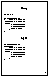To illustrate this problem, consider the following program for computing the fibonacci function.

```int s1, s2 ;

int fibonacci (int n)
{
if (n == 0) return 1;
else if (n == 1) return 1;
else {
s1 = fibonacci(n-1);
s2 = fibonacci(n-2);
return s1 + s2;
}
}```

The main thing to note here is that the variables that will hold the intermediate results, S1 and S2, have been declared as global variables. This is a mistake. Although the function looks just fine, its correctness crucially depends on having local variables for storing all the intermediate results. As shown, it will not correctly compute the fibonacci function for n=4 or larger. However, if we move the declaration of s1 and s2 inside the function, it works perfectly.

This sort of bug is very hard to find, and bugs like this are almost certain to arise whenever you use global variables to store intermediate results of a recursive function.

This sort of bug does not seem to arise if iteration is used instead of recursion. However, this is not an argument against recursion: it is an argument against using global variables.You should always follow this rule: Always declare variables in the function where they are needed.Now, let us return to the question of efficiency. Recursion is based upon calling the same function over and over, whereas iteration simply `jumps back' to the beginning of the loop. A function call is often more expensive than a jump. The overheads that may be associated with a function call are:

Space:
Every invocation of a function call may require space for parameters and local variables, and for an indication of where to return when the function is finished. Typically this space (allocation record) is allocated on the stack and is released automatically when the function returns. Thus, a recursive algorithm may need space proportional to the number of nested calls to the same function.

Time:
The operations involved in calling a function - allocating, and later releasing, local memory, copying values into the local memory for the parameters, branching to/returning from the function - all contribute to the time overhead.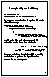If a function has very large local memory requirements, it would be very costly to program it recursively. But even if there is very little overhead in a single function call, recursive functions often call themselves many many times, which can magnify a small individual overhead into a very large cumulative overhead. Consider, for example, the factorial function

```int factorial(int n)
{
if (n == 0) return 1;
else        return n * factorial(n-1);
}```
There is very little overhead in calling this function, as it has only one word of local memory, for the parameter n. However, when we try to compute factorial(20), there will end up being 21 words of memory allocated - one for each invocation of the function:
```factorial(20) -- allocate 1 word of memory,
call factorial(19) -- allocate 1 word of memory,
call factorial(18) -- allocate 1 word of memory,
.
.
.
call factorial(2) -- allocate 1 word of memory,
call factorial(1) -- allocate 1 word of memory,
call factorial(0) -- allocate 1 word of memory,```
at this point 21 words of memory and 21 activation records have been allocated.
```                             return 1.         -- release  1 word of memory.

return 1*1.       -- release  1 word of memory.
return 2*1.       -- release  1 word of memory.```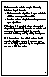The other reason that recursive programs may turn out to be inefficient is the simple fact that, when we use recursion to solve problems we are interested exclusively with correctness, and not at all with efficiency. Consequently, our simple, elegant recursive algorithms may be inherently inefficient.Therefore, it is essential, after we have solved the problem, to critically examine our solution and attempt to improve on it; both by removing sources of inefficiency and by refining its elegance.

The fibonacci algorithm is a very striking example: if we directly coded it in C it is an exponential algorithm, meaning that in order to compute fibonacci(N) it would require about 2**N additions. Yet there is a very simple iterative version that requires only about N additions to compute fibonacci(N). This algorithm too can be expressed as a straightforward recursive computation.

Therefore, the lesson is not that recursion is intrinsically inefficient, but rather that there are efficient algorithms as well as inefficient ones. We need to be able to analyze our algorithms to get a ballpark estimate of their efficiency. We can directly implement any of the recursive algorithms that are reasonably efficient. We will learn how to this analysis later in the course (it's the next chapter in the text).

One final note about programming using recursion. I mentioned that most programming languages permit recursive function calls, but you might occasionally be using a language that does not. This does not mean that there is no way to use recursive algorithms.

Most recursive algorithms can be translated, by a fairly mechanical procedure, into iterative algorithms. Sometimes this is very straightforward - for example, most compilers detect a special form of recursion, called tail recursion, and automatically translate into iteration without your knowing. Sometimes, the translation is more involved: for example, it might require introducing an explicit stack with which to `fake' the effect of recursive calls.